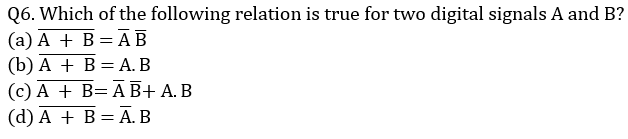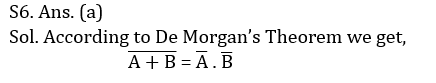Engineering Jobs   »   Electrical Engineering quizs   »   UPPSC Polytechnic Lecturer Eligibility Criteria, UPPSC...

# UPPSC-LECTURER’21 EE: Daily Practices Quiz 05-Oct-2021

## UPPSC Technical Education Teaching Exam Notification 2021

Uttar Pradesh Public Service Commission has released the official notification for the recruitment of PrincipalLecturerLibrarian, and Workshop Superintendent. The Online application has been started on 15th September 2021 and the last date to apply online is 15th October 2021. The recruitment is for a total of 1370 UPPSC Polytechnic Lecturer Vacancies.

## UPPSC-LECTURER’21 EE:  Daily Practices Quiz

UPPSC-LECTURER’21 EE: Daily Practices Quiz 05-Oct-2021

Each question carries 3 marks.
Negative marking: 1 mark each or 1/3rd
Total Questions: 06
Time: 08 min.

Q1. Which of the following is a non-maskable interrupt of 8085 microprocessors?
(a) INTR
(b) TRAP
(c) RST 6.5
(d) RST 7.5

Q2. A 1:5 step-up transformer has 120 V across the primary and 600 Ω resistance across the secondary. Assuming, 100 % efficiency, the primary current equals…
(a) 5 A
(b) 10 A
(c) 20 A
(d) 0.2 A

Q3. A bipolar transistor has α=0.98, ICO=10 µA. If the base current is 100 µA, then collector current would be
(a) 4.9 mA
(b) 2.9 mA
(c) 3.4 mA
(d) 5.4 mA

Q4. What are the average and RMS values of half wave rectified output if peak voltage is 5 V?
(a) 1.59 V, 2.5 V
(b) 3.18 V, 2.5 V
(c) 1.59 V, 5 V
(d) 3.18 V, 5 V

Q5. What is the value of charging current in short transmission lines?
(a) Less than medium transmission lines
(b) Equal to medium transmission lines
(c) More than medium transmission lines
(d) More than long transmission lines## SOLUTIONS

S1. Ans.(b)
Sol. The hardware vectored interrupts are classified in to maskable and non-maskable interrupts.
RST 7.5, RST 6.5, RST 5.5 and INTR are maskable interrupt.

S2. Ans.(a)
Sol. K=5
R1=600/K^2 =600/25=24 Ω
∴I1=V1/R1 =120/24=5 A

S3. Ans.(d)
Sol. α=0.98 (given)
∴current gain factor(β)=α/(1-α)=0.98/(1-0.98)=49
∴IC=βIB+(1+β) ICO=49×100×10^(-6)+(1+49)×10×10^(-6)=5.4 mA

S4. Ans.(a)
Sol. For half-wave rectifier: Vavg=Vm/π=5/3.14=1.59 V
And Vrms=Vm/2=5/2=2.5 V

S5. Ans.(a)
Sol. Line to Earth capacitance of short transmission line is less than that in medium and long transmission line and is negligible. Because of this the charging current of short transmission line is less than that in medium and long transmission line and is negligible.Sharing is caring!

Thank You, Your details have been submitted we will get back to you.